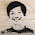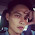## 2014年1月21日 星期二

### MathJax : 在網頁中顯示數學式子

$$\frac{1}{5}+\frac{2}{5}=\frac{3}{5}$$

＄＄\frac{1}{5}+\frac{2}{5}=\frac{3}{5}＄＄

<script type="text/javascript">
window.MathJax = {
jax: ["input/TeX","output/HTML-CSS"],
extensions: ["tex2jax.js"],
tex2jax: {
inlineMath: [['＄','＄'],['\$','\$']]
},
};
</script>

<script src="http://cdn.mathjax.org/mathjax/latest/MathJax.js" type="text/javascript"></script>

http://cdn.mathjax.org/mathjax/latest/MathJax.js

https://c328740.ssl.cf1.rackcdn.com/mathjax/latest/MathJax.js

$$\frac{1}{5}$$

#### 6 則留言:

1.網路上有很多在google小工具設定後就能使用的經驗分享，但在下試了都失敗，只有您的作法成功。

特別想請問的是，假如在下使用的是google bloger，是否有辦法只要一次的設定，之後在文章編輯器時就能直接用Mathjax的語法撰寫呢？

目前在下使用您的方法，就是每次打文章前，都要到文章的htlm貼上您所提供的code才行，希望能夠更簡單些，期待能獲得您的解惑，感謝您！！

1.您好:
Blogger 可以自訂範本(template)，將語法加入範本的 HTML 語法中應該可以讓每篇文章都能使用，缺點是即使沒有使用的到 MathJax 也會載入 JavaScript 程式，應該會拖顯示頁面的速度。

2.謝謝您！已成功解決^ ^

3.2.不好意思，我想用Blogger 寫數學式，但用了很多方法都失敗，包誇您提供的做法，實在不知道哪裡出了問題。
可以請問會是因為版本瀏覽器版本有差嗎還是還有其他可能？

1.您好:
是不是瀏覽器的問題, 您自己試了便可以知道答案囉~~
個人在 Chrome 和 Firefox 中是可以成功的，IE 的話,您就別浪費時間了。

© 2009. Design by Pocket